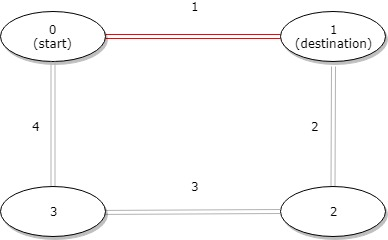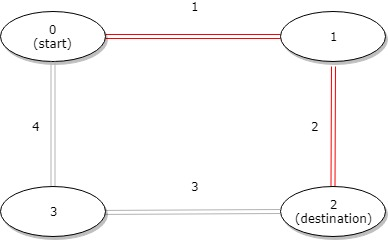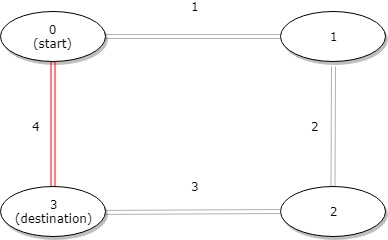1184. Distance Between Bus Stops

#### QUESTION:

A bus has n stops numbered from 0 to n - 1 that form a circle. We know the distance between all pairs of neighboring stops where distance[i] is the distance between the stops number i and (i + 1) % n.

The bus goes along both directions i.e. clockwise and counterclockwise.

Return the shortest distance between the given start and destination stops.

Example 1:Input: distance = [1,2,3,4], start = 0, destination = 1 Output: 1 Explanation: Distance between 0 and 1 is 1 or 9, minimum is 1.

Example 2:Input: distance = [1,2,3,4], start = 0, destination = 2 Output: 3 Explanation: Distance between 0 and 2 is 3 or 7, minimum is 3.

Example 3:Input: distance = [1,2,3,4], start = 0, destination = 3 Output: 4 Explanation: Distance between 0 and 3 is 6 or 4, minimum is 4.

Constraints:

1 <= n <= 10^4 distance.length == n 0 <= start, destination < n 0 <= distance[i] <= 10^4

#### EXPLANATION:

1.循环遍历，从start开始，因为start计算的是start->start+1的距离，所以需要加上
2.循环到destination，因为destination是destination->destination+1的距离，所以不需要加上
3.这样我们就计算出来了之间的距离a
4.另外一个距离就是num-a
5.计算两者的小的返回结果

#### SOLUTION:

``````class Solution {
public int distanceBetweenBusStops(int[] distance, int start, int destination) {
int clock = 0;
int counterclock = 0;
int min = Math.min(start,destination);
int max = Math.max(start,destination);
for(int i = 0;i<distance.length;i++){
if(i<min || i>=max){
clock+=distance[i];
}else{
counterclock+=distance[i];
}
}
return Math.min(counterclock,clock);
}
}
``````
>